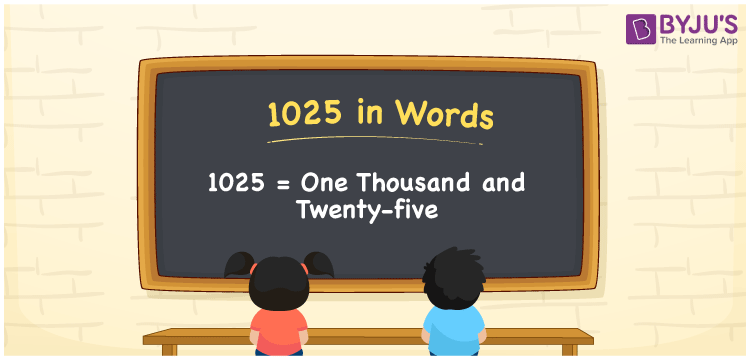# 1025 in Words

We can write 1025 in words as One Thousand and Twenty-five. Suppose you bought a new wall clock that costs Rs. 1025, then you could say that “ I bought a new wall clock for One Thousand and Twenty-five rupees”. In this article, you will learn how to convert the number 1025 into words in an exciting way. The number 1025 is used in expressions that relate to money, days, distance, length, weight and so on.

 1025 in words One Thousand and Twenty-five One Thousand and Twenty-five in Numerals 1025

## 1025 in English Words

We generally write numbers in words using the English alphabet. Thus, we can read 1025 in English as “One Thousand and Twenty-five”.## How to Write 1025 in Words?

In this section, you will learn how to write the number 1025 in words. The given number 1025 has four digits, so we should make a place value chart that represents the place value for all these four digits. The order of place values of digits in a number is in accordance with the Indian numbering system, such as

• Ones
• Tens
• Hundreds
• Thousands
• Ten-thousands
• Hundred-thousands or Lakhs

This can be done as follows.

 Thousands Hundreds Tens Ones 1 0 2 5

Thus, we can write the expanded form as:

1 × Thousand + 0 × Hundred + 2 × Ten + 5 × One

= 1 × 1000 + 0 × 100 + 2 × 10 + 5 × 1

= 1025

= One thousand twenty-five.

1025 is a natural number that precedes 1026 and succeeds 1024.

1025 in words – One Thousand and Twenty-five

Is 1025 an odd number? – Yes

Is 1025 an even number? – No

Is 1025 a perfect square number? – No

Is 1025 a perfect cube number? – No

Is 1025 a prime number? – No

Is 1025 a composite number? – Yes

## Frequently Asked Questions on 1025 in Words

Q1

### How do you write 1025 in English?

We can write 1025 in English words as “One Thousand and Twenty-five”.
Q2

### How do you write 1025 in words for a cheque?

For a cheque, we generally write 1025 in words as One Thousand and Twenty-five rupees only.
Q3

### What number is 1025 in words?

The number 1025 is One Thousand and Twenty-five in words.
Test your Knowledge on 1025 in Words